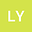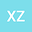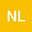Two-step discretization method for 2D/3D Allen-Cahn equation base on RBF-FD scheme
•••• Lin Yao,
• Xindong Zhang,
• Ning Li
Lin Yao
Xinjiang Normal University

Corresponding Author:[email protected]

Author ProfileXindong Zhang
Xinjiang Normal University
Author ProfileNing Li
Henan Agricultural University
Author Profile## Abstract

Based on the multi-quadrics (MQ) radial basis function, the two-step discretization method will be used to solve Allen-Cahn (AC) equation in this paper. In the first step, we will use the Backward Euler Formula to solve the AC equation with Newton iterative, and then apply the global RBF method to solve the Semi discrete linearized problem on a small number of collocation points or on a coarse mesh. In the second step, finite difference (FD) and radial basis function-finite difference (RBF-FD) methods will be used to solve the problem on a fine mesh or on a large number of collocation points, respectively. The two-step method will be proved to be more feasible and efficient by some numerical examples. In addition, compared with FD method, the application of RBF-FD method will improve the accuracy of the numerical solution in the second step.